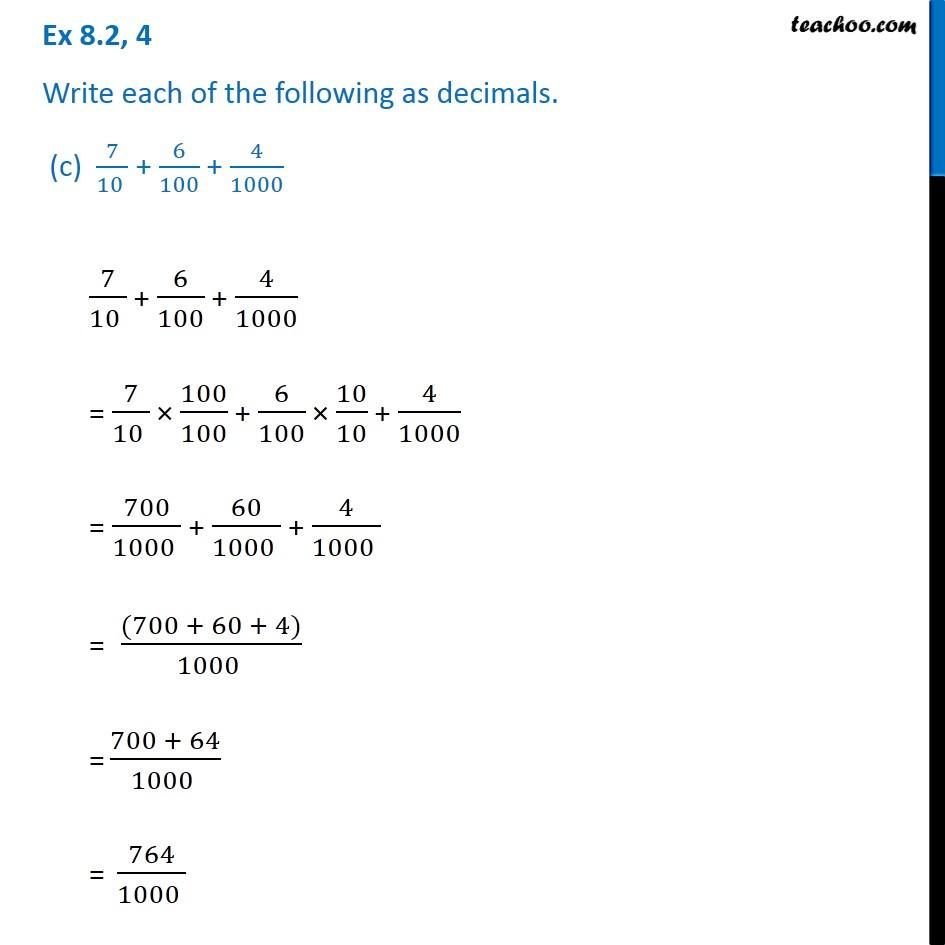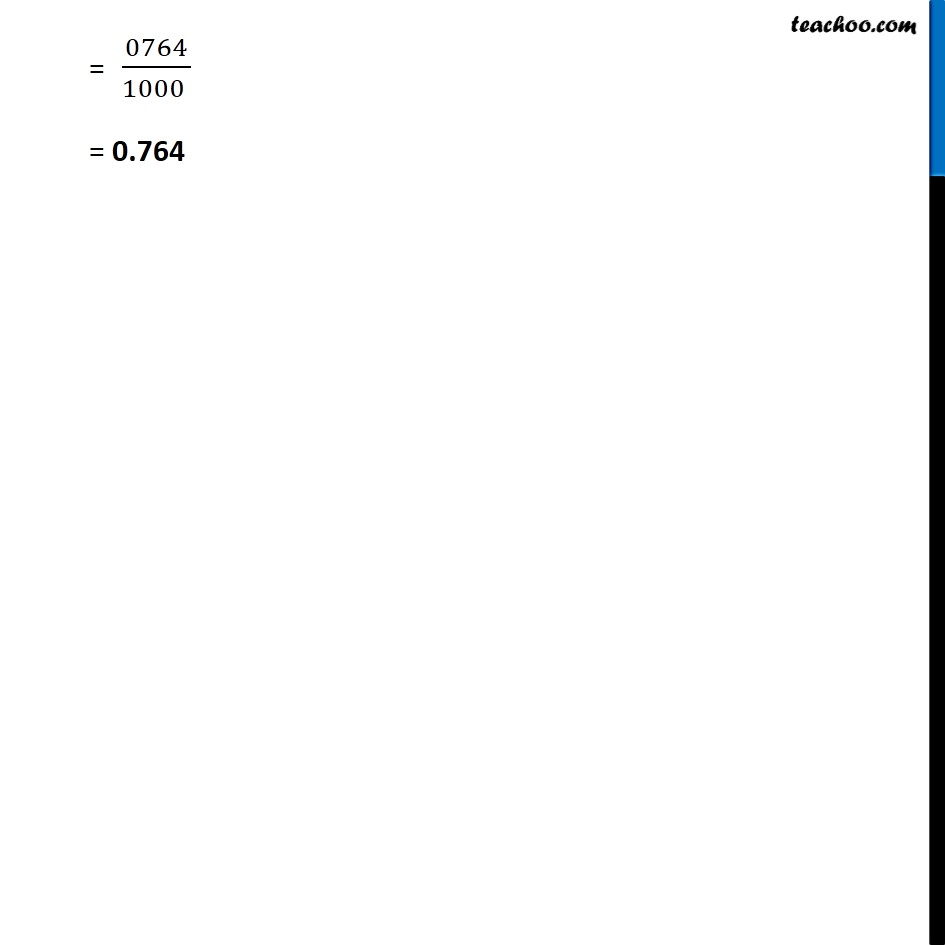Ex 8.2

Chapter 8 Class 6 Decimals
Serial order wiseGet live Maths 1-on-1 Classs - Class 6 to 12

### Transcript

Ex 8.2, 4 Write each of the following as decimals. (c) 7/(10 ) + 6/100 + 4/1000 7/(10 ) + 6/100 + 4/1000 = 7/(10 ) 100/100 + 6/100 10/10 + 4/1000 = 700/(1000 ) + 60/(1000 ) + 4/(1000 ) = ((700 + 60 + 4))/(1000 ) = (700 + 64)/(1000 ) = 764/(1000 ) = 0764/(1000 ) = 0.764